Date

How do you tackle something as complex as understanding how to create an interpreter or compiler? In the beginning it all looks pretty much like a tangled mess of yarn that you need to untangle to get that perfect ball.

The way to get there is to just untangle it one thread, one knot at a time. Sometimes, though, you might feel like you don’t understand something right away, but you have to keep going. It will eventually “click” if you’re persistent enough, I promise you (Gee, if I put aside 25 cents every time I didn’t understand something right away I would have become rich a long time ago :).

Probably one of the best pieces of advice I could give you on your way to understanding how to create an interpreter and compiler is to read the explanations in the articles, read the code, and then write code yourself, and even write the same code several times over a period of time to make the material and code feel natural to you, and only then move on to learn new topics. Do not rush, just slow down and take your time to understand the basic ideas deeply. This approach, while seemingly slow, will pay off down the road. Trust me.

You will eventually get your perfect ball of yarn in the end. And, you know what? Even if it is not that perfect it is still better than the alternative, which is to do nothing and not learn the topic or quickly skim over it and forget it in a couple of days.

Remember - just keep untangling: one thread, one knot at a time and practice what you’ve learned by writing code, a lot of it: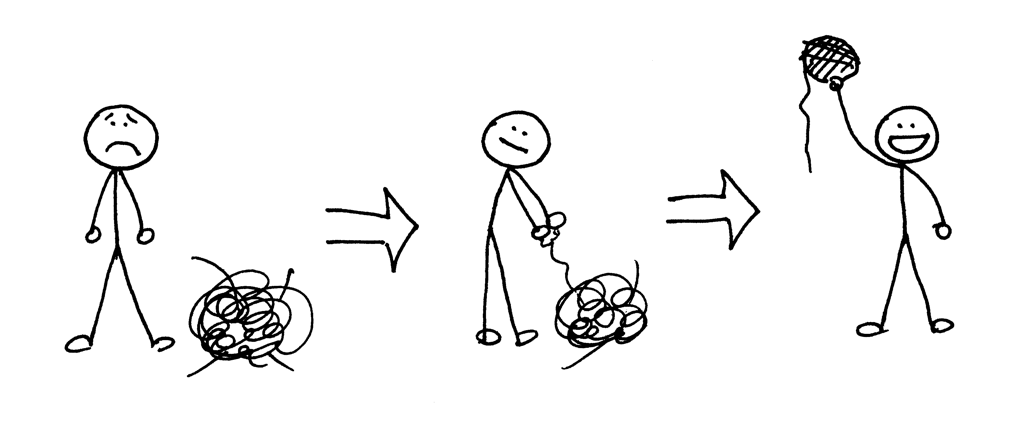Today you’re going to use all the knowledge you’ve gained from previous articles in the series and learn how to parse and interpret arithmetic expressions that have any number of addition, subtraction, multiplication, and division operators. You will write an interpreter that will be able to evaluate expressions like “14 + 2 * 3 - 6 / 2”.

Before diving in and writing some code let’s talk about the associativity and precedence of operators.

By convention 7 + 3 + 1 is the same as (7 + 3) + 1 and 7 - 3 - 1 is equivalent to (7 - 3) - 1. No surprises here. We all learned that at some point and have been taking it for granted since then. If we treated 7 - 3 - 1 as 7 - (3 - 1) the result would be unexpected 5 instead of the expected 3.

In ordinary arithmetic and most programming languages addition, subtraction, multiplication, and division are left-associative:

```7 + 3 + 1 is equivalent to (7 + 3) + 1
7 - 3 - 1 is equivalent to (7 - 3) - 1
8 * 4 * 2 is equivalent to (8 * 4) * 2
8 / 4 / 2 is equivalent to (8 / 4) / 2
```

What does it mean for an operator to be left-associative?

When an operand like 3 in the expression 7 + 3 + 1 has plus signs on both sides, we need a convention to decide which operator applies to 3. Is it the one to the left or the one to the right of the operand 3? The operator + associates to the left because an operand that has plus signs on both sides belongs to the operator to its left and so we say that the operator + is left-associative. That’s why 7 + 3 + 1 is equivalent to (7 + 3) + 1 by the associativity convention.

Okay, what about an expression like 7 + 5 * 2 where we have different kinds of operators on both sides of the operand 5? Is the expression equivalent to 7 + (5 * 2) or (7 + 5) * 2? How do we resolve this ambiguity?

In this case, the associativity convention is of no help to us because it applies only to operators of one kind, either additive (+, -) or multiplicative (*, /). We need another convention to resolve the ambiguity when we have different kinds of operators in the same expression. We need a convention that defines relative precedence of operators.

And here it is: we say that if the operator * takes its operands before + does, then it has higher precedence. In the arithmetic that we know and use, multiplication and division have higher precedence than addition and subtraction. As a result the expression 7 + 5 * 2 is equivalent to 7 + (5 * 2) and the expression 7 - 8 / 4 is equivalent to 7 - (8 / 4).

In a case where we have an expression with operators that have the same precedence, we just use the associativity convention and execute the operators from left to right:

```7 + 3 - 1 is equivalent to (7 + 3) - 1
8 / 4 * 2 is equivalent to (8 / 4) * 2
```

I hope you didn’t think I wanted to bore you to death by talking so much about the associativity and precedence of operators. The nice thing about those conventions is that we can construct a grammar for arithmetic expressions from a table that shows the associativity and precedence of arithmetic operators. Then, we can translate the grammar into code by following the guidelines I outlined in Part 4, and our interpreter will be able to handle the precedence of operators in addition to associativity.

Okay, here is our precedence table: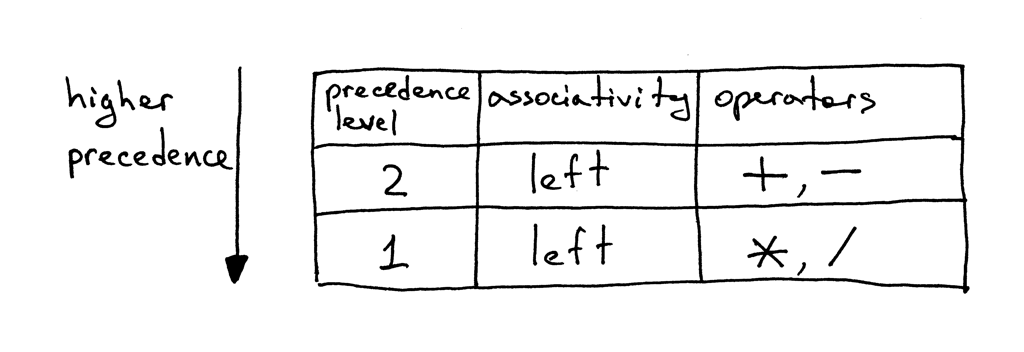From the table, you can tell that operators + and - have the same precedence level and they are both left-associative. You can also see that operators * and / are also left-associative, have the same precedence among themselves but have higher-precedence than addition and subtraction operators.

Here are the rules for how to construct a grammar from the precedence table:

1. For each level of precedence define a non-terminal. The body of a production for the non-terminal should contain arithmetic operators from that level and non-terminals for the next higher level of precedence.
2. Create an additional non-terminal factor for basic units of expression, in our case, integers. The general rule is that if you have N levels of precedence, you will need N + 1 non-terminals in total: one non-terminal for each level plus one non-terminal for basic units of expression.

Onward!

Let’s follow the rules and construct our grammar.

According to Rule 1 we will define two non-terminals: a non-terminal called expr for level 2 and a non-terminal called term for level 1. And by following Rule 2 we will define a factor non-terminal for basic units of arithmetic expressions, integers.

The start symbol of our new grammar will be expr and the expr production will contain a body representing the use of operators from level 2, which in our case are operators + and - , and will contain term non-terminals for the next higher level of precedence, level 1:The term production will have a body representing the use of operators from level 1, which are operators * and / , and it will contain the non-terminal factor for the basic units of expression, integers:And the production for the non-terminal factor will be:You’ve already seen above productions as part of grammars and syntax diagrams from previous articles, but here we combine them into one grammar that takes care of the associativity and the precedence of operators: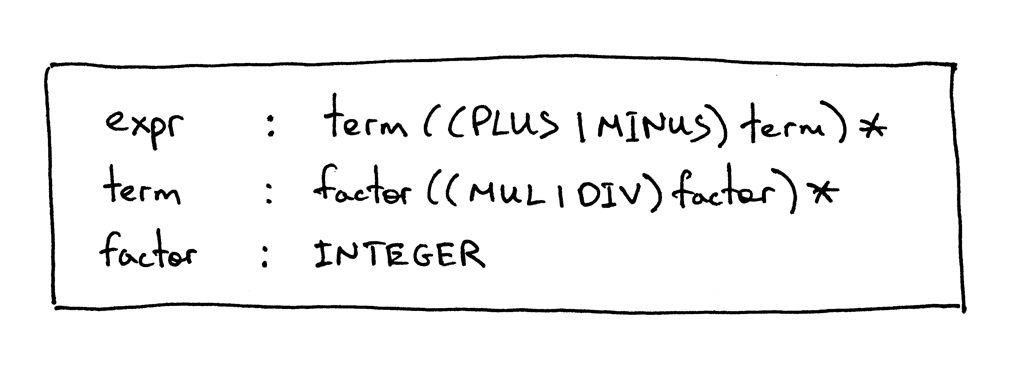Here is a syntax diagram that corresponds to the grammar above: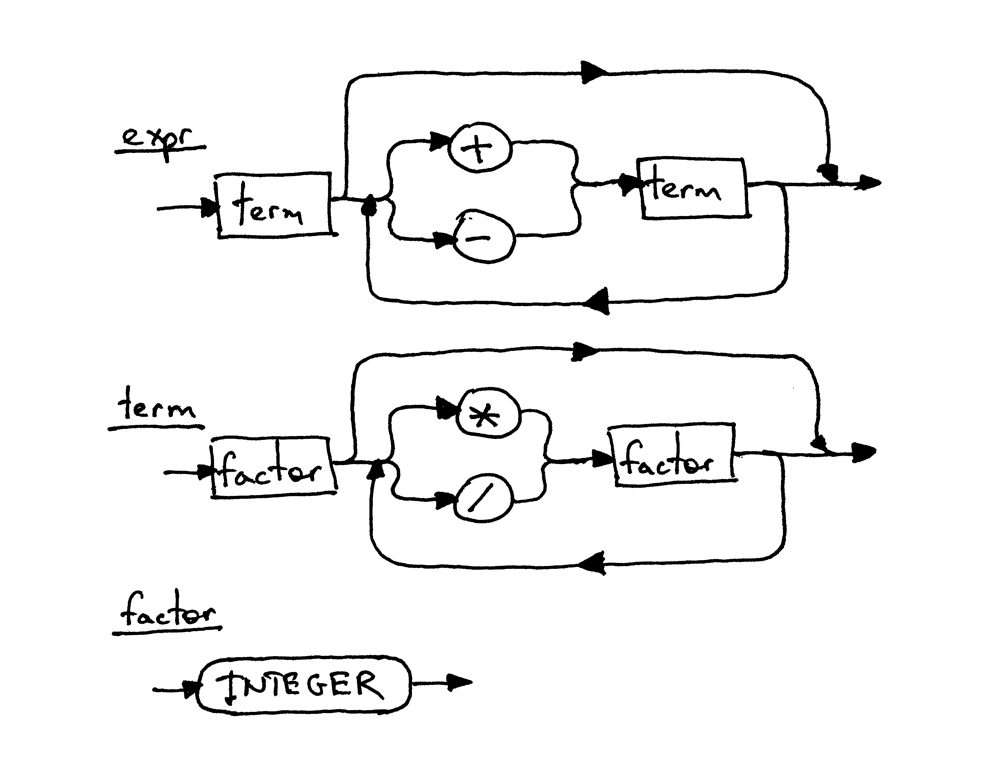Each rectangular box in the diagram is a “method call” to another diagram. If you take the expression 7 + 5 * 2 and start with the top diagram expr and walk your way down to the bottommost diagram factor, you should be able to see that higher-precedence operators * and / in the lower diagram execute before operators + and - in the higher diagram.

To drive the precedence of operators point home, let’s take a look at the decomposition of the same arithmetic expression 7 + 5 * 2 done in accordance with our grammar and syntax diagrams above. This is just another way to show that higher-precedence operators execute before operators with lower precedence: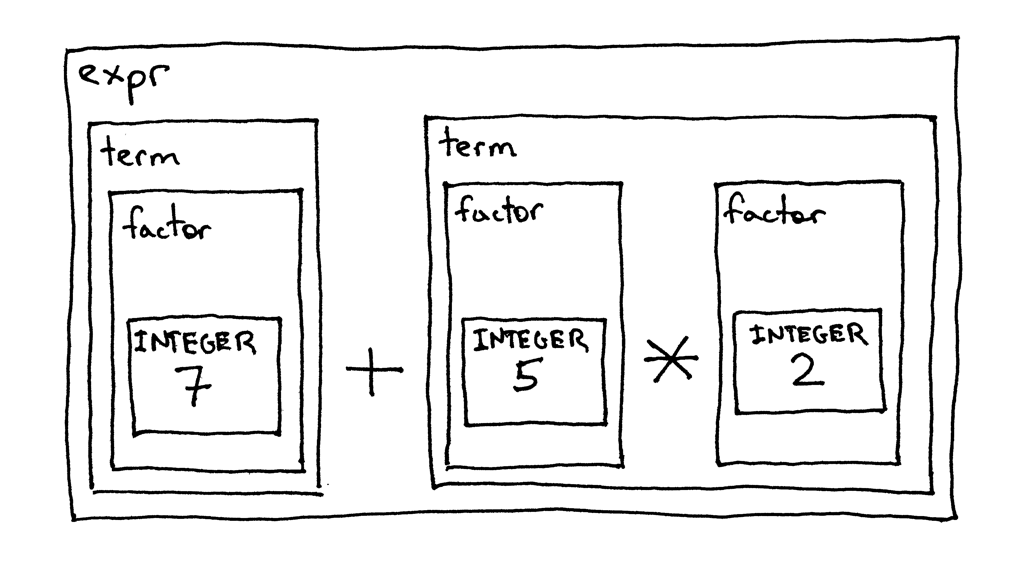Okay, let’s convert the grammar to code following guidelines from Part 4 and see how our new interpreter works, shall we?

Here is the grammar again:And here is the complete code of a calculator that can handle valid arithmetic expressions containing integers and any number of addition, subtraction, multiplication, and division operators.

The following are the main changes compared with the code from Part 4:

• The Lexer class can now tokenize +, -, *, and / (Nothing new here, we just combined code from previous articles into one class that supports all those tokens)
• Recall that each rule (production), R, defined in the grammar, becomes a method with the same name, and references to that rule become a method call: R(). As a result the Interpreter class now has three methods that correspond to non-terminals in the grammar: expr, term, and factor.

Source code:

```# Token types
#
# EOF (end-of-file) token is used to indicate that
# there is no more input left for lexical analysis
INTEGER, PLUS, MINUS, MUL, DIV, EOF = (
'INTEGER', 'PLUS', 'MINUS', 'MUL', 'DIV', 'EOF'
)

class Token(object):
def __init__(self, type, value):
# token type: INTEGER, PLUS, MINUS, MUL, DIV, or EOF
self.type = type
# token value: non-negative integer value, '+', '-', '*', '/', or None
self.value = value

def __str__(self):
"""String representation of the class instance.

Examples:
Token(INTEGER, 3)
Token(PLUS, '+')
Token(MUL, '*')
"""
return 'Token({type}, {value})'.format(
type=self.type,
value=repr(self.value)
)

def __repr__(self):
return self.__str__()

class Lexer(object):
def __init__(self, text):
# client string input, e.g. "3 * 5", "12 / 3 * 4", etc
self.text = text
# self.pos is an index into self.text
self.pos = 0
self.current_char = self.text[self.pos]

def error(self):
raise Exception('Invalid character')

"""Advance the `pos` pointer and set the `current_char` variable."""
self.pos += 1
if self.pos > len(self.text) - 1:
self.current_char = None  # Indicates end of input
else:
self.current_char = self.text[self.pos]

def skip_whitespace(self):
while self.current_char is not None and self.current_char.isspace():

def integer(self):
"""Return a (multidigit) integer consumed from the input."""
result = ''
while self.current_char is not None and self.current_char.isdigit():
result += self.current_char
return int(result)

def get_next_token(self):
"""Lexical analyzer (also known as scanner or tokenizer)

This method is responsible for breaking a sentence
apart into tokens. One token at a time.
"""
while self.current_char is not None:

if self.current_char.isspace():
self.skip_whitespace()
continue

if self.current_char.isdigit():

if self.current_char == '+':

if self.current_char == '-':

if self.current_char == '*':

if self.current_char == '/':

self.error()

class Interpreter(object):
def __init__(self, lexer):
self.lexer = lexer
# set current token to the first token taken from the input
self.current_token = self.lexer.get_next_token()

def error(self):
raise Exception('Invalid syntax')

def eat(self, token_type):
# compare the current token type with the passed token
# type and if they match then "eat" the current token
# and assign the next token to the self.current_token,
# otherwise raise an exception.
if self.current_token.type == token_type:
self.current_token = self.lexer.get_next_token()
else:
self.error()

def factor(self):
"""factor : INTEGER"""
token = self.current_token
self.eat(INTEGER)

def term(self):
"""term : factor ((MUL | DIV) factor)*"""
result = self.factor()

while self.current_token.type in (MUL, DIV):
token = self.current_token
if token.type == MUL:
self.eat(MUL)
result = result * self.factor()
elif token.type == DIV:
self.eat(DIV)
result = result / self.factor()

return result

def expr(self):
"""Arithmetic expression parser / interpreter.

calc>  14 + 2 * 3 - 6 / 2
17

expr   : term ((PLUS | MINUS) term)*
term   : factor ((MUL | DIV) factor)*
factor : INTEGER
"""
result = self.term()

while self.current_token.type in (PLUS, MINUS):
token = self.current_token
if token.type == PLUS:
self.eat(PLUS)
result = result + self.term()
elif token.type == MINUS:
self.eat(MINUS)
result = result - self.term()

return result

def main():
while True:
try:
# To run under Python3 replace 'raw_input' call
# with 'input'
text = raw_input('calc> ')
except EOFError:
break
if not text:
continue
lexer = Lexer(text)
interpreter = Interpreter(lexer)
result = interpreter.expr()
print(result)

if __name__ == '__main__':
main()
```

Save the above code into the calc5.py file or download it directly from GitHub. As usual, try it out and see for yourself that the interpreter properly evaluates arithmetic expressions that have operators with different precedence.

Here is a sample session on my laptop:

```\$ python calc5.py
calc> 3
3
calc> 2 + 7 * 4
30
calc> 7 - 8 / 4
5
calc> 14 + 2 * 3 - 6 / 2
17
```

Here are new exercises for today:• Write an interpreter as described in this article off the top of your head, without peeking into the code from the article. Write some tests for your interpreter, and make sure they pass.

• Extend the interpreter to handle arithmetic expressions containing parentheses so that your interpreter could evaluate deeply nested arithmetic expressions like: 7 + 3 * (10 / (12 / (3 + 1) - 1))

Check your understanding.

1. What does it mean for an operator to be left-associative?
2. Are operators + and - left-associative or right-associative? What about * and / ?
3. Does operator + have higher precedence than operator * ?

Hey, you read all the way to the end! That’s really great. I’ll be back next time with a new article - stay tuned, be brilliant, and, as usual, don’t forget to do the exercises.

Here is a list of books I recommend that will help you in your study of interpreters and compilers:

1. Language Implementation Patterns: Create Your Own Domain-Specific and General Programming Languages (Pragmatic Programmers)2. Writing Compilers and Interpreters: A Software Engineering Approach3. Modern Compiler Implementation in Java4. Modern Compiler Design5. Compilers: Principles, Techniques, and Tools (2nd Edition)